## Example Questions

### Example Question #5 : How To Add Fractions

What is the result of addingofto?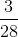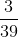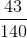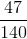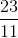Explanation:

Let us first get our value for the percentage of the first fraction. 20% of 2/7 is found by multiplying 2/7 by 2/10 (or, simplified, 1/5): (2/7) * (1/5) = (2/35)

Our addition is therefore (2/35) + (1/4). There are no common factors, so the least common denominator will be 35 * 4 or 140. Multiply the numerator and denominator of 2/35 by 4/4 and the numerator of 1/4 by 35/35.

This yields:

(8/140) + (35/140)  = 43/140, which cannot be reduced.

### Example Question #1 : How To Add Fractions

Reduce to simplest form: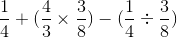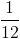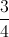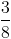Explanation:

Simplify expressions inside parentheses first: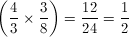and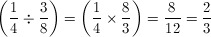Now we have: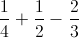Add them by finding the common denominator (LCM of 4, 2, and 3 = 12) and then multiplying the top and bottom of each fraction by whichever factors are missing from this common denominator: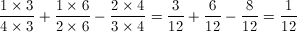### Example Question #2 : How To Add Fractions

Quantity A: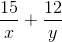Quantity B: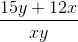Which of the following is true?

Quantity A is larger.

The relationship between the two quantities cannot be determined.

The two quantities are equal.

Quantity B is larger.

The two quantities are equal.

Explanation:

Start by looking at Quantity A. The common denominator for this expression is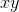. To calculate this, you perform the following multiplications: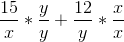This is the same as: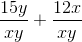, orThis is the same as Quantity B. They are equal!

Tired of practice problems?

Try live online GRE prep today.